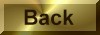Practice Problem 8

Predict whether the following reaction is still spontaneous at 500°C:

N2(g) + 3 H2(g)2 NH3(g)

Assume that the values ofHo andS° used in Practice Problem 7 are still valid at this temperature.

Solution

Before we can decide whether the reaction is still spontaneous we need to calculate the temperature of the kelvin scale:

TK = 500o C + 273 = 773 K

We then multiply the entropy term by this temperature and subtract this quantity from the enthalpy term:Go773 =Ho298 - TSo298

= 92,220 J - (773 K x -198.75 J/K)

= 92,220 J - (-153,600 J)

= 61,380 JGo = 61.4 kJ

Because the entropy term becomes larger as the temperature increases, the reaction changes from one which is favorable at low temperatures to one that is unfavorable at high temperatures.